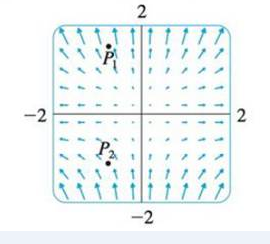Chapter 16.9, Problem 20E

Chapter
Section
Textbook Problem

(a) Are the points P1 and P2 sources or sinks for the vector field F shown in the figure? Give an explanation based solely on the picture.(b) Given that F(x, y) = ⟨x, y2⟩, use the definition of divergence to verify your answer to part (a).(a)

To determine

Whether the points P1 and P2 are sources or sinks for the vector field F .

Explanation

If the net flow of the vectors is outward at a point P in the vector field, then the divergence of the vector field at the point P is positive and the point is called source.

If the net flow of the vectors is inward at a point P in the vector field, then the divergence of the vector field at the point P is negative and the point is called sink.

Refer to Figure in the textbook (i.e., bottom of the question 16.9-20E).

From the Figure in the textbook, the vectors enter towards the point P1 are shorter than the vectors leaving from the point P1 .

As the vectors that end near the point P1 are shorter than the vectors that start near the point P1 , the net flow of the vectors is outward.

Therefore, the divergence of the vector field is positive and the point P1 is called as source

(b)

To determine

To verify: The answers in Part (a) with the use of definition of divergence.

Still sussing out bartleby?

Check out a sample textbook solution.

See a sample solution

The Solution to Your Study Problems

Bartleby provides explanations to thousands of textbook problems written by our experts, many with advanced degrees!

Get Started

22. Write as a difference involving two binomials.

Mathematical Applications for the Management, Life, and Social Sciences

True or False: If f(c) = 0, then c is a point of inflection for f.

Study Guide for Stewart's Single Variable Calculus: Early Transcendentals, 8th

True or false:

Study Guide for Stewart's Multivariable Calculus, 8th